# Community

Blog Events Webinars Tutorials Forum
×
Community Blog The Clever Design of Java Map

# The Clever Design of Java Map

This article classifies and summarizes some of the source codes and several core features of Java Map based on the author's understanding.

By ZiyunRecently, the author has reviewed some source codes for Java Map. The design ideas of these developers are astonishing and can be referenced for practices. However, most introduction articles on the principles of Java Map focus on separated issues instead of the principle network. Therefore, based on the author's understanding, this article classifies and summarizes some of the source codes and several core features of Java Map, including automatic scaling, initialization and lazy load, hash calculation, bit operation, and concurrency. The author hopes this article can help readers in understanding and practicing. In this article, HashMap in JDK 1.8 is used by default.

## 1. Automatic Scaling

• Minimum Viable Principle: The capacity is scaled automatically when it exceeds a certain threshold.

The `resize()` method is used to scale the capacity. Scale-out is performed at the end of the `putVal` method after elements are written. After elements are written, if the size is greater than the previously calculated threshold, the resize() method runs.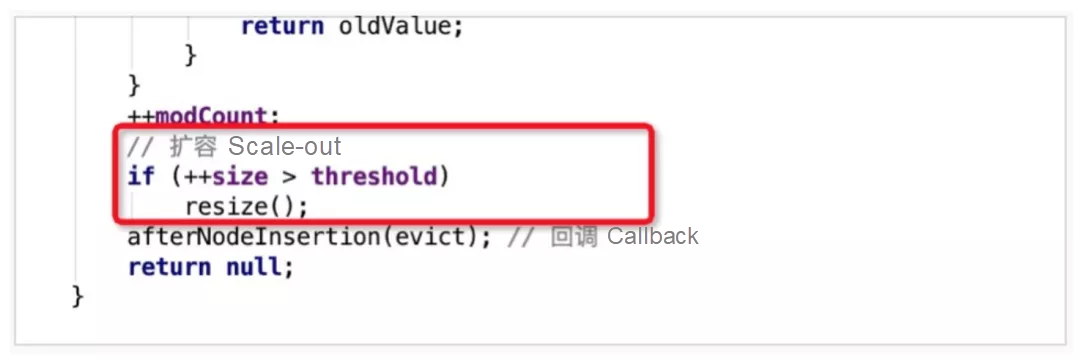The capacity is scaled using the bitwise operation "<< 1", which means the capacity is doubled. The new threshold newThr is also doubled.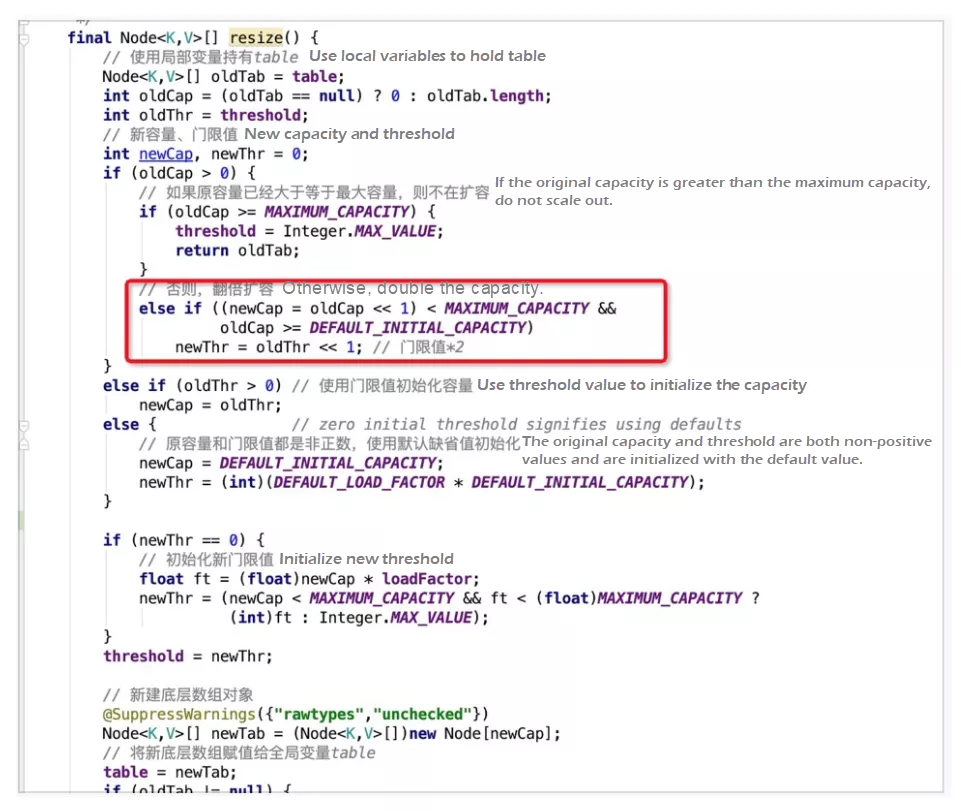Three scenarios exist for scale-out:

• If only one element is found in a certain position in the hash bucket array, then no hash conflict exists. Copy the element to the corresponding position in the new hash bucket array
• If a node in a specified position in the hash bucket array is a tree node, the corresponding red-black tree (R-B tree) is scaled out.
• If a node in a certain position in the hash bucket array is a normal node, the linked list is scaled out. In JDK 1.8, to avoid the dead chain problem caused by concurrent scale-out in earlier JDK versions, it introduces high-bit and low-bit linked lists to assist with the scale-out operation.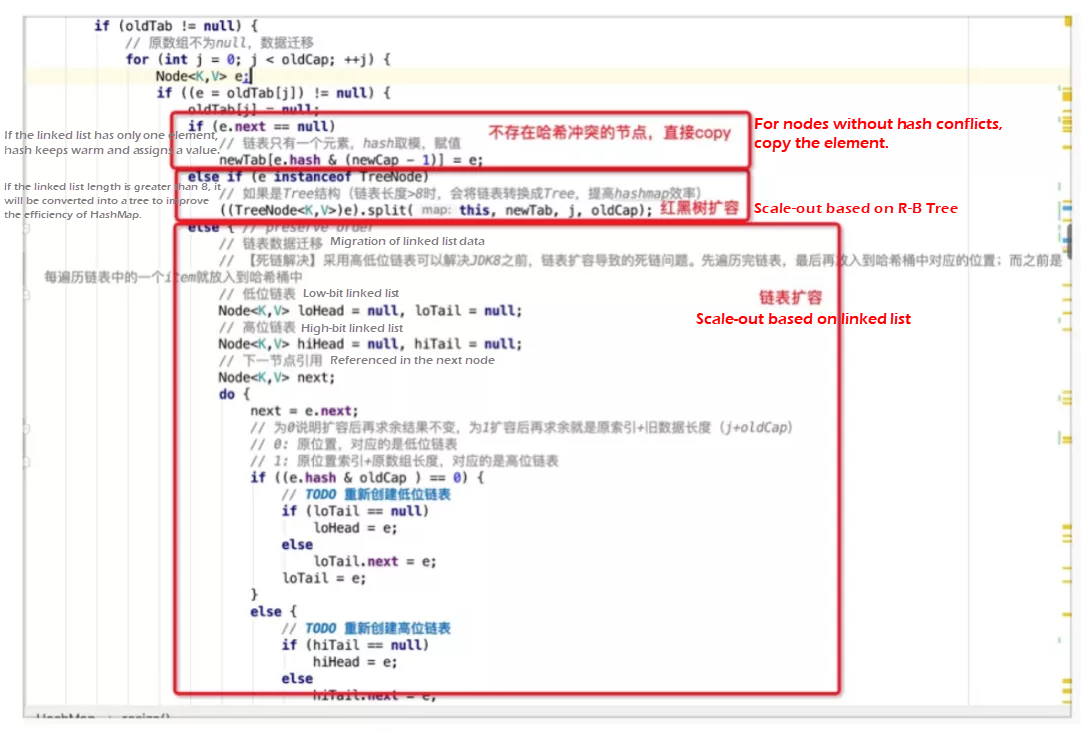In daily development, developers may encounter some bad cases, for example:

``````HashMap hashMap = new HashMap(2);
hashMap.put("1", 1);
hashMap.put("2", 2);
hashMap.put("3", 3);``````

When HashMap sets the last element 3, it will find that the size of the current hash bucket array has reached the threshold of 2 x 0.75 = 1.5. So, it performs the scale-out operation. This code performs the scale-out operation every time it is run, which is inefficient. In daily development, the size of HashMap must be properly decided to make sure that the scale-out threshold is greater than the number of stored elements to reduce the number of scale-out operations.

## 2. Initialization and Lazy Load

The default load factor is only set during initialization. Other initialization operations for the factor are performed when it is used for the first time.

When a new HashMap is created, the hash array is not initialized immediately. Instead, it is initialized using the `resize()` method when the element is put for the first time.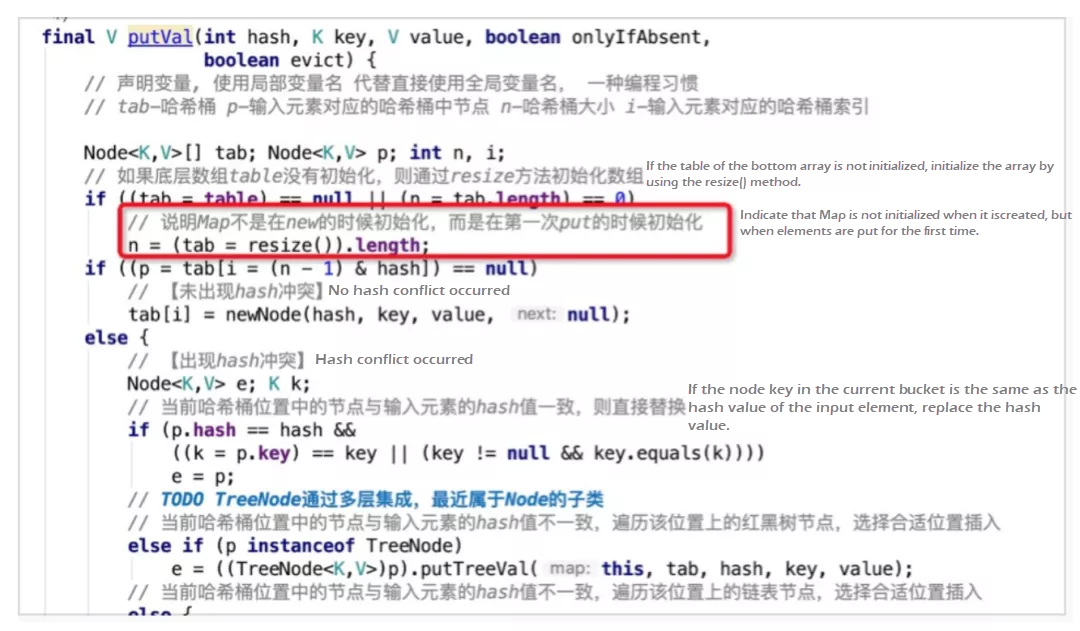The default capacity `DEFAULT_INITIAL_CAPACITY` is set to 16 using the `resize()` method, and the scaling threshold is 0.75 x 16 = 12. When the number of elements in the hash bucket array reaches 12, the capacity is scaled out.

A node array with a capacity of 16 is created, and the member variable hash bucket table is assigned with a value. Thus, the HashMap initialization is completed.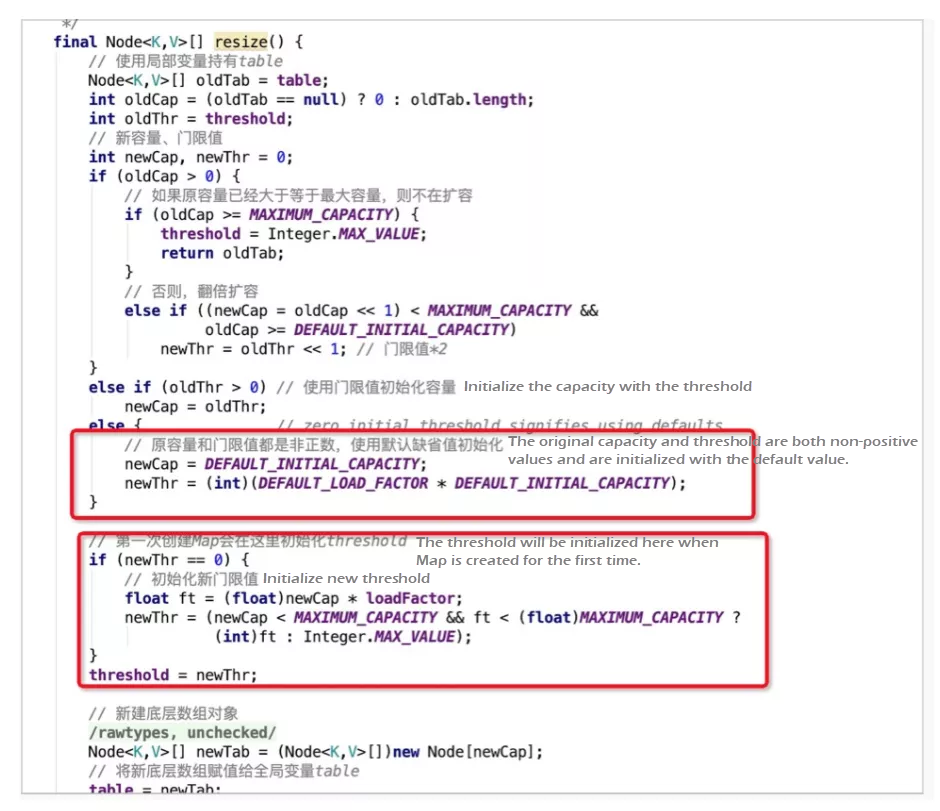## 3. Hash Value Calculation

The name hash table demonstrates the importance of hash value calculation in the data structure. However, JDK does not use the `hashCode` returned by the native method of the Object as the final hash value to realize hash value calculation. Instead, it performs secondary processing.

The following examples show how `HashMap` and `ConcurrentHashMap` calculate the hash value. The core logic is the same. They use `hashCode` corresponding to a key, and the result after `hashCode` shifts to the right by 16 bits for the XOR operation. Here, the code that performs XOR operation on the 16-bit high and 16-bit low `hashCode` is called a disturbing function. It aims to integrate the features of high-bit `hashCode` into the low-bit `hashCode` and reduce the probability of hash conflict.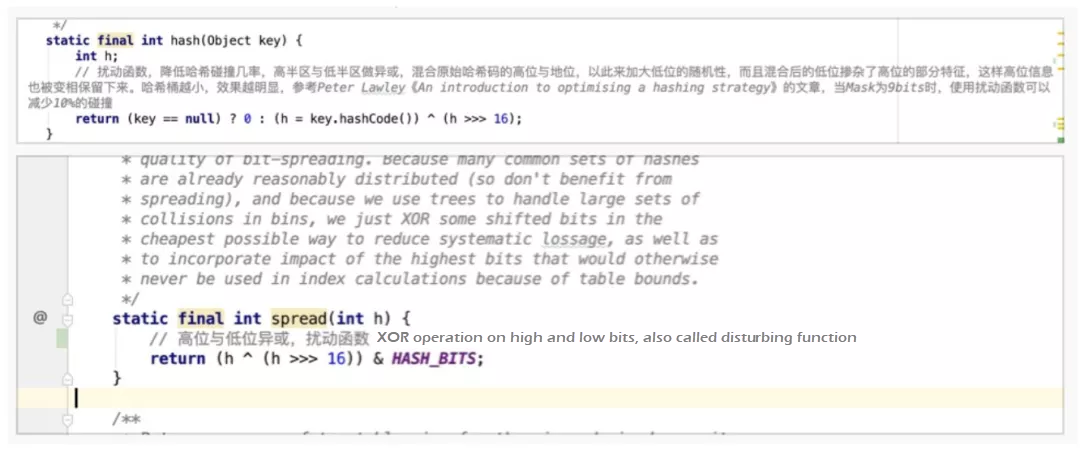Here is an example of the disturbing function:

``````hashCode(key1) = 0000 0000 0000 1111 0000 0000 0000 0010
hashCode(key2) = 0000 0000 0000 0000 0000 0000 0000 0010``````

If the `HashMap` capacity is 4, the `hashCode` of key1 conflicts with key2 if the disturbing function is not used. The latter two bits are the same, which are 10.

If the disturbing function is used, the last two bits of `hashCode` become different, so the hash conflict can be avoided.

``````hashCode(key1) ^ (hashCode(key1) >>> 16)
0000 0000 0000 1111 0000 0000 0000 1101

hashCode(key2) ^ (hashCode(key2) >>> 16)
0000 0000 0000 0000 0000 0000 0000 0010``````

The benefit of disturbing function increases as the HashMap capacity decreases. In the article entitled An Introduction to Optimising a Hashing Strategy, 352 strings with different hash values are randomly selected. When the `HashMap` capacity is 2^9 , the disturbing function can reduce 10% of hash conflicts. Therefore, the disturbing function is necessary.

In addition, after the hashCode is processed by the disturbing function in `ConcurrentHashMap`, the AND operation is required between the `hashCode` and `HASH_BITS. HASH_BITS is 0 x 7ffffff`. Only the highest bit is 0, so the `hashCode` after the operation is always positive. `ConcurrentHashMap` defines the `hashCode` of several special nodes, such as MOVED, TREEBIN, and RESERVED. The `hashCode` values of these nodes are negative. Therefore, setting the `hashCode` of a common node to a positive value will not conflict with the `hashCode` of special nodes.

### 3.1 Hash Conflict

The hash value calculation can map different input values to specified value ranges, but hash conflicts may occur. Assume that all input elements are mapped to the same hash bucket after hash value calculation. The query complexity is no longer O(1), but O(n), which is equivalent to the sequential traversal of the linear table. Therefore, hash conflict is one of the important factors that affect the performance of hash value calculation. The way to resolve hash conflict involves two aspects. One solution is to avoid conflicts, and the other is to resolve conflicts properly to maximize query efficiency. The former aspect has been introduced in the section above where the disturbing function is used to increase the randomness of `hashCode` to avoid conflicts. For the latter aspect, HashMap provides two solutions: linked list pulling and R-B tree.

On versions earlier than JDK 1.8, `HashMap` resolves hash conflicts by pulling linked lists. For example, the bucket corresponding to the calculated `hashCode` already contains elements with different keys. At this time, a linked list is pulled out based on the existing elements in the bucket, and the new elements are linked in front of the existing elements. When the hash bucket contains conflict is queried, elements in the conflicting chain are traversed sequentially. The following figure shows the logic to identify the same key. First, check whether the hash values are the same. Then, compare whether the addresses or values of keys are the same.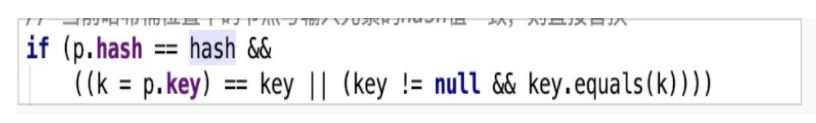On versions earlier than JDK 1.8, a bug that causes a dead chain exists in `HashMap` during concurrent scale-out. An endless loop is formed when getting an element at that position, and the CPU utilization remains high. This also shows that `HashMap` is not suitable for high concurrency scenarios. In this kind of scenario, `ConcurrentHashMap` in `java.util.concurrent` (JUC) is recommended. However, JDK developers chose to face the bug directly and solve it. In JDK 1.8, high-bit and low-bit linked lists (double-end linked lists) are introduced.

What are high-bit and low-bit linked lists? During scale-out, the bucket capacity of the hash bucket array will be doubled. For example, for a `HashMap` with the capacity of 8, the capacity will be scaled out to 16. [0, 7] bits are low bits, and [8, 15] bits are high bits. The low bit corresponds to loHead and loTail, and the high bit corresponds to hiHead and hiTail.

During scale-out, the elements of each position in the old bucket array are traversed in sequence.

• If no conflicts occur, perform MOD operation on hash values again and copy the elements to the corresponding position in the new bucket array.
• If conflicting elements exist, the high-bit and low-bit linked lists are used for processing. Run the `e.hash & oldCap` statement to determine whether the value is in high bits or low bits after the MOD operation is performed. For example, if the hashCode value of the current element is 0001 (ignoring the high bits), the operation result is equal to 0. It means the result after scale-out remains unchanged, and the value after MOD operation is still in low bits [0, 7], 0001 & 1000 = 0000. Then, use the low-bit linked list to link this type of element. If the `hashCode` value of the current element is 1001, the result of the operation is not 0, 1001 & 1000 = 1000. After scale-out, the value is still in the high bits, and the new position just happens to be 9, which is the sum of the old array index (1) and old data length (8). Then, use the high-bit linked list to link these elements. Finally, put the head nodes of the high-bit and low-bit linked lists to the specified positions in the array `newTab` respectively after scale-out. This solution reduces the access frequency to the shared resource newTab and avoids the dead chain problem caused by concurrent scale-out in versions earlier than JDK 1.8, where `newTab` is accessed each time an element is traversed.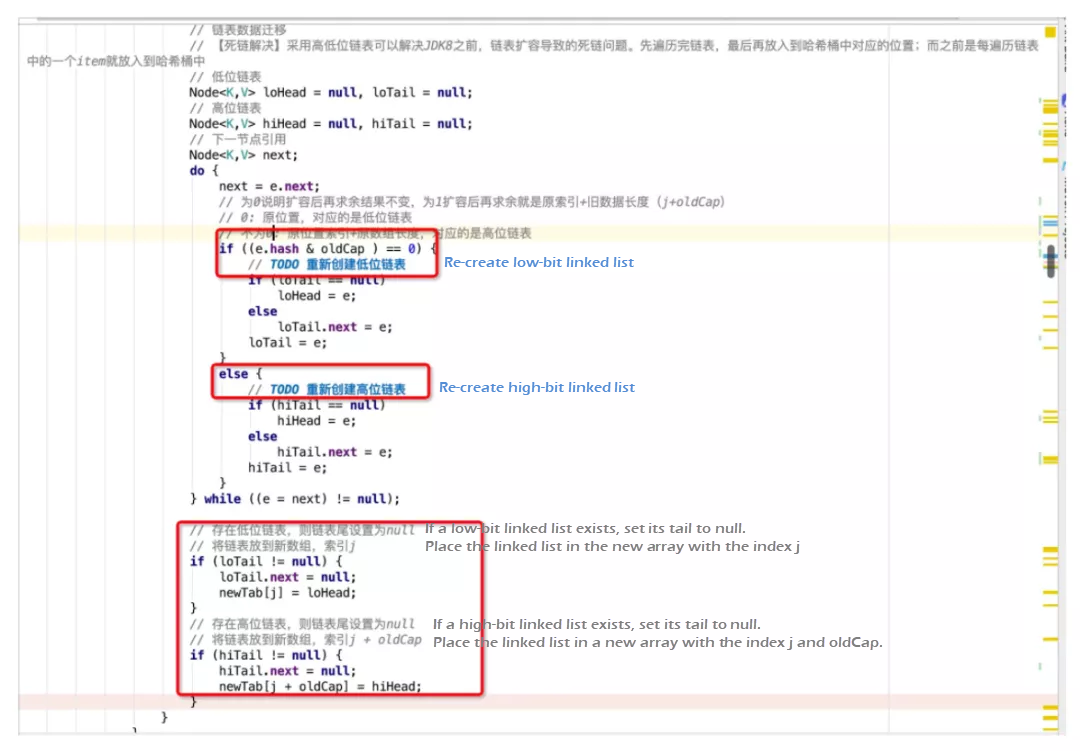#### R-B Tree

In JDK 1.8, HashMap introduces thr R-B tree to deal with hash conflicts, instead of pulling linked lists. Why is the R-B tree introduced to replace linked lists? Although the insertion performance of the linked list is O(1), the query performance is O(n). If hash conflicts occur among multiple elements, the query performance is unacceptable. Therefore, in JDK 1.8, if the number of elements in the conflict link is greater than 8, and the length of the hash bucket array is greater than 64, the R-B tree will be used instead of the linked list to resolve the hash conflict. The nodes will be encapsulated as `TreeNodes` instead of Nodes to achieve the query performance of O(logn). `TreeNodes` inherit the Nodes to utilize the polymorphism feature.

Here is a brief introduction to the R-B tree. It is a balanced binary search tree that is similar to the Adelson-Velsky and Landis (AVL) tree. The key difference between them is that the AVL tree focuses on absolute balance, resulting in higher cost when adding or deleting nodes. However, AVL tree provides better query performance and applies to scenarios with more reads than writes. For HashMap, read and write operations are nearly the same in number. Therefore, the R-B tree provides balanced read and write performance.

## 4. Bitwise Operation

### 4.1 Determine the Size of Hash Bucket Array

Find the smallest integer power of 2 that is greater than or equal to the set value. `tableSizeFor` returns the final capacity of the hash bucket array based on the input capacity cap and finds the smallest integer power of 2 that is greater than or equal to the given value of cap. At first glance, this row-by-row bitwise operation is confusing, but we can understand it by finding the pattern.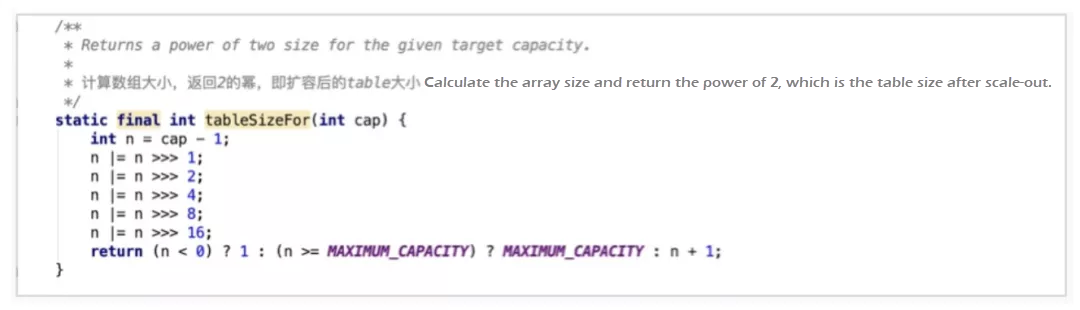When cap equals 3, the calculation process works like this:

``````cap = 3
n = 2
n |= n >>> 1       010  | 001 = 011   n = 3
n |= n >>> 2       011  | 000 = 011   n = 3
n |= n >>> 4       011  | 000 = 011   n = 3
….
n = n + 1 = 4``````

When cap equals 5, the calculation process works like this:

``````cap = 5
n = 4
n |= n >>> 1    0100 | 0010 = 0110  n = 6
n |= n >>> 2    0110 | 0001 = 0111  n = 7
….
n = n + 1 = 8``````

Therefore, the calculation aims to find the smallest integer power of 2 that is greater than or equal to the value of cap. The entire process is to find 1 of the highest bits in the binary system corresponding to cap and shift it by 1, 2, 4, 8, and 16 bits at a step size of 2^n 2n each time. Copy 1 of the highest bit to all subsequent low bits, set all bits after 1 of the highest bit to 1, and add 1 to them to complete the carry.

The change process of sample binary bits is listed below:

``````0100 1010
0111 1111
1000 0000``````

This method can be regarded as the minimum viable principle (MVP), which means the space is occupied as little as possible. The question is, why must it be an integer power of 2? This requirement can improve the calculation and storage efficiency and ensure that the hash value of each element can accurately be within the specified range of the hash bucket array. The algorithm used to determine the array subscript is `hash & (n - 1)`, where n is the size of the hash bucket array. n is always an integer power of 2. So, the binary form of n - 1 is always 0000111111, and multiple digits of 1 appear continuously starting from the lowest bit. The result of the `& operation` on this binary value and any value is mapped to the specified range [0, n - 1]. For example, if n = 8, the binary value corresponding to "n – 1" is 0111. After the `& operation`, the result is always within the range of [0, 7]. In other words, the result is in the given eight hash buckets, and the storage space utilization rate is 100%. For a counter-example, when n = 7, the corresponding binary value of "n – 1" is 0110. The result of the `& operation` is in No. 0, No. 6, No. 4, and No. 2 hash buckets instead of the range [0, 6]. The No. 1, No. 3, and No. 5 buckets are skipped, which leads to a storage space utilization rate of less than 60%. At the same time, hash conflicts are more prone to occur.

### 4.2. Calculate ASHIFT Offset

Obtain the highest effective bits of a given value. In addition to multiplication and division, the shift can retain high and low bits. The right shift retains high bits and the left shift retains low bits.

The ABASE and ASHIFT in `ConcurrentHashMap` calculate the initial position of an element in the hash array in the actual memory. ASHIFT subtracts the number of leading 0 of scale from the number 31; it subtracts 1 from the actual number of digits in scale. "scale" is the size of each element in the hash bucket array Node[]. The initial position of the No. i element in the array in the memory is obtained using the `((long)i << ASHIFT) + ABASE)` expression.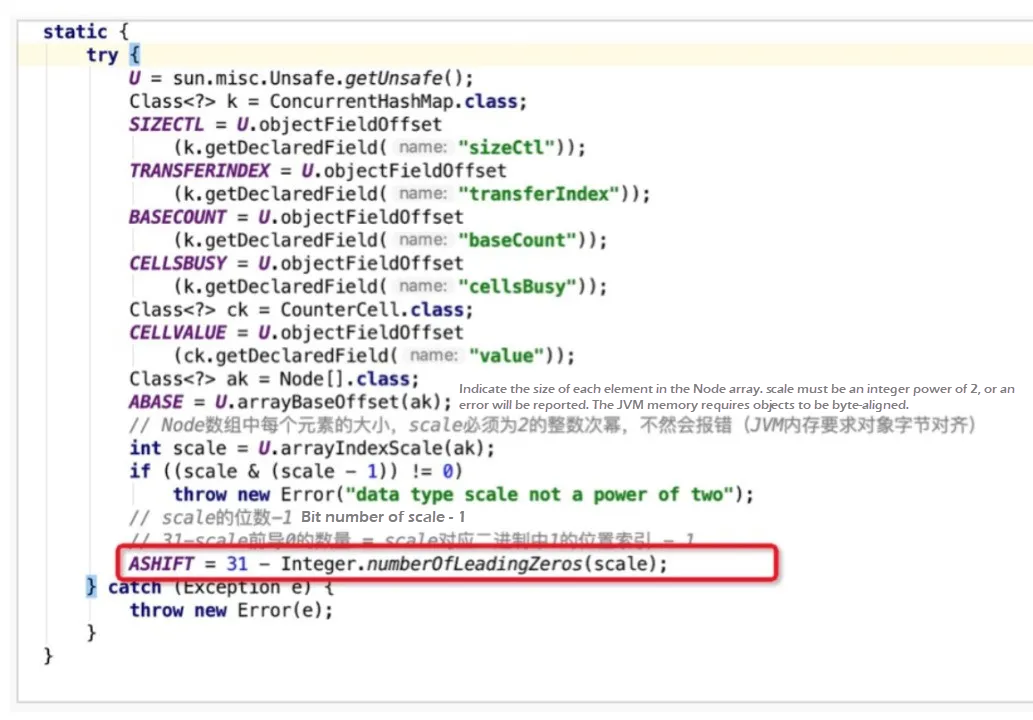Let's see how the number of leading zeros is calculated. `numberOfLeaderingZeros` is an Integer static method. It is easier to understand this by finding the pattern.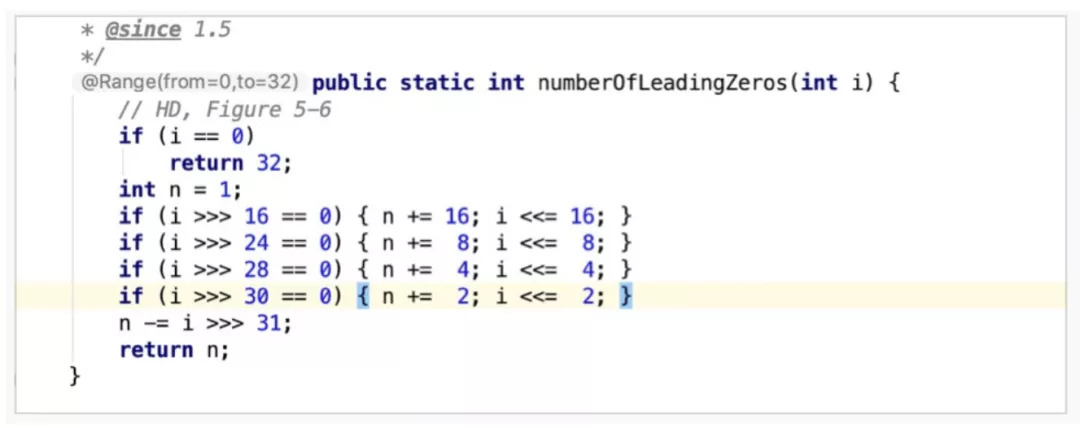Assume that i = 0000 0000 0000 0100 0000 0000 0000 0000 and n = 1:

``````i >>> 16  0000 0000 0000 0000 0000 0000 0000 0100   Not all are 0

i >>> 24  0000 0000 0000 0000 0000 0000 0000 0000   All are 0``````

All bits are 0 after the right shifts by 24 bits, indicating that the digits between the 24th bit and 31st bit must all be 0; then, n = 1 + 8 = 9. The highest eight bits are all 0 and the information has been recorded in n. Therefore, these eight bits can be discarded, which means that i is shifted to the left by eight bits.

``i = 0000 0100 0000 0000 0000 0000 0000 0000``

Similarly, all bits of i are 0 after the right shifts by 28 bits, which means the digits between the 28th bit and 31st bits are all 0. Then, n = 9 + 4 = 13, and the highest four bits are discarded.

``i = 0100 0000 0000 0000 0000 0000 0000 0000``

Continue to calculate

``````i >>> 30  0000 0000 0000 0000 0000 0000 0000 0001   Not all are 0
i >>> 31  0000 0000 0000 0000 0000 0000 0000 0000   All are 0``````

Finally, the conclusion of n = 13 is obtained, which means there exist 13 leading zeros. The right shift of i by 31 bits aims to check whether the highest 31st bit is 1 because the initial bit is 1. If it is 1, no leading zero exists, which means n = n - 1 = 0.

In conclusion, the operation above is based on the idea of dichotomy to locate the highest bit of 1 in the binary value. First, check the high 16 bits. If all bits are 0, it means that 1 exists in the low 16 bits. If not all bits are 0, 1 exists in the high 16 bits. By doing so, the search range is reduced from 32 bits (31 bits to be exact) to 16 bits. Perform search operation in this pattern until 1 is found.

The numbers of bits checked during calculation are 16, 8, 4, 2, and 1, respectively. The total number is 31. Why is it 31, not 32? When the number of leading zeros is 32, i can only be 0 and is filtered by the "if" condition. So, the leading zeros can only appear at the highest 31 bits to be checked. The number of highest effective bits can be calculated by subtracting the number of leading zeros from the total number of bits. In the code, the statement is 31 - `Integer.numberOfLeaderingZeros(scale)`, where the total number of bits is not 32. This is to obtain the initial position of the No. i element in the hash bucket array in the memory, which facilitates compare and swap (CAS) and other operations.

## 5. Concurrency

### 5.1 Pessimistic Lock

#### Full-Table Lock

`HashTable` adopts full-table lock, which means all operations are locked and executed in sequence. The `put()` method in the following figure is an example and is modified by a keyword, synchronized. Although this solution ensures thread security, it also affects the calculation performance significantly in the era of multi-core processors. This is why `HashTable` has been abandoned by developers gradually.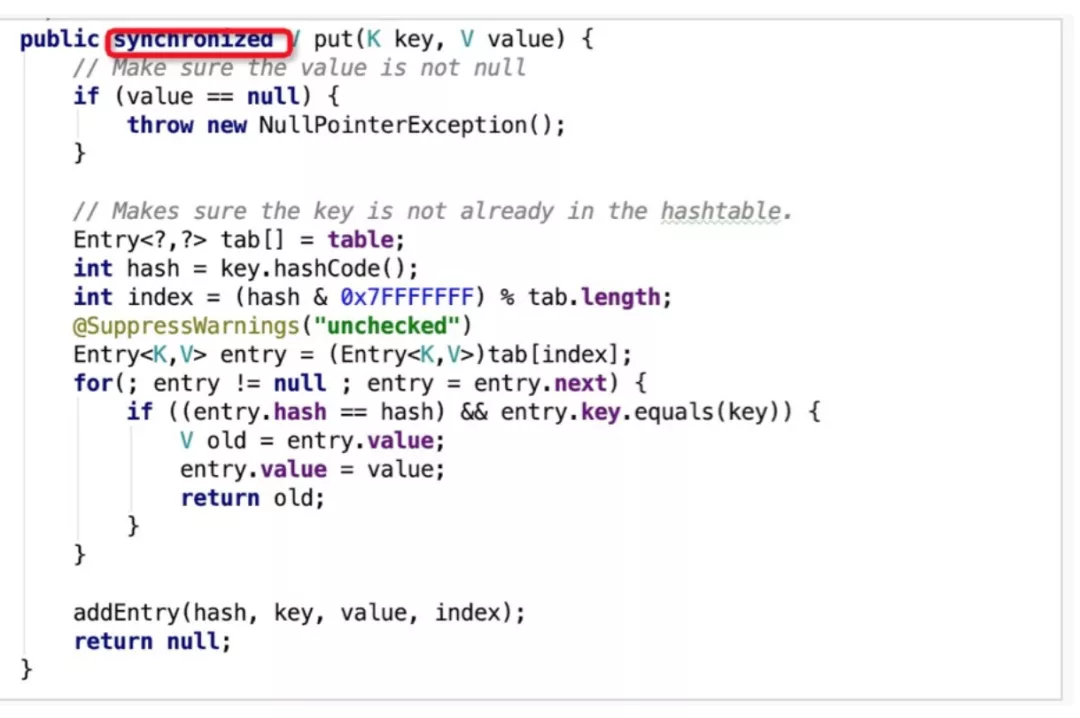#### Segment Lock

`ConcurrentHashMap` introduced the segment lock mechanism before JDK 1.8 to address problems saying the lock granularity in `HashTable` was too coarse. The following figure shows the overall storage structure. Multiple segments are split based on the original structure, and each segment is mounted with an original entry (the hash bucket array frequently mentioned above.) During each operation, lock the segment where the element is located instead of the entire table. Therefore, the locked range is smaller, so the concurrency is higher.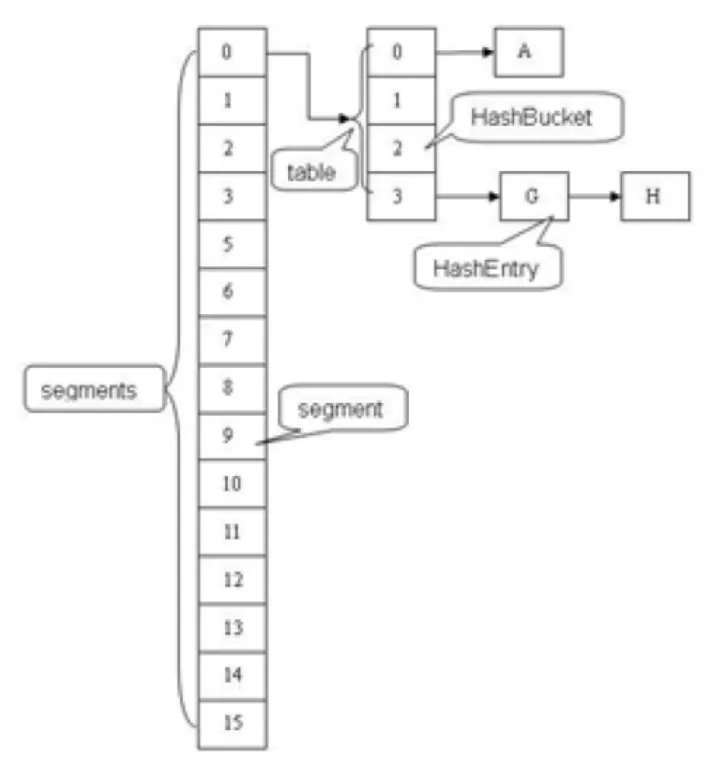### 5.2 Optimistic Lock

#### Synchronized + CAS

The segment lock mechanism can ensure thread security and solve the problem of low performance caused by coarse lock granularity. For engineers pursuing ultimate performance, segment lock is not the perfect solution. Therefore, in JDK 1.8, `ConcurrentHashMap` abandons the segment lock and uses the optimistic lock. The main reasons for abandoning the segment lock are listed below:

• Segments occupy the storage space of `ConcurrentHashMap`.
• When one segment is too large, the concurrency performance drops sharply.

In JDK 1.8, `ConcurrentHashMap` abandons the segment structure and uses the Node array structure similar to `HashMap`.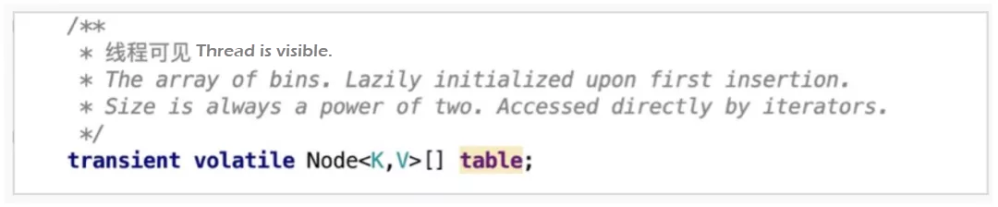The optimistic lock in `ConcurrentHashMap` is implemented using the keyword synchronized and CAS operation. Let's take a look at the code related to the `put()` method.

If the element to be put does not exist in the hash bucket array, the CAS operation is performed.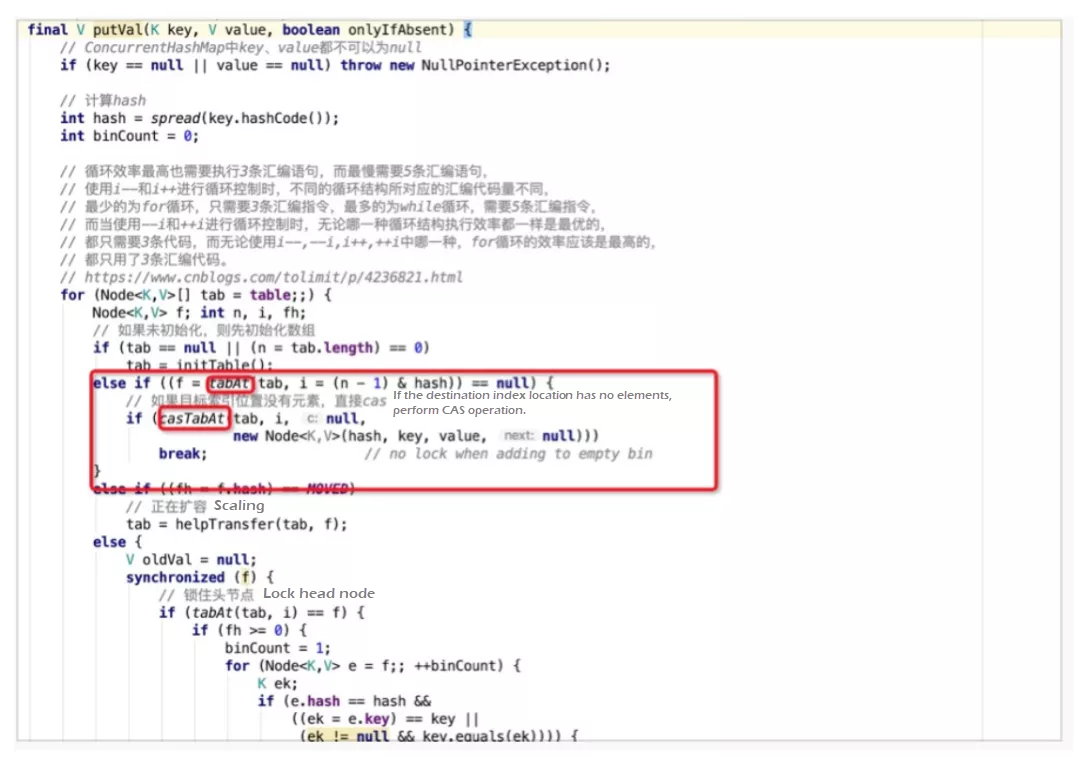Two important operations are involved, namely, `tabAt` and `casTabAt`. In the code above, the Unsafe class is used. The Unsafe class is rarely used in the daily development process. Developers always think Java is safe to use because all operations are performed on Java Virtual Machine (JVM) and are secured and controlled. However, the Unsafe class breaks the safe conditions and enables Java to work like the C language. With this class, Java can operate any memory addresses, so it is a double-edged sword. In the code above, ASHIFT calculates the initial memory address of the specified element. Then, `getObjectVolatile` and `compareAndSwapObject` obtains the values and performs CAS operations.

Why is `getObjectVolatile` used instead of the `get()` method to obtain the element at the specified position in the hash bucket array? In the JVM memory model, each thread has its own working memory, which is the local variable table in the stack. The working memory is a copy of the main memory. Therefore, thread 1 updates a shared resource and writes it to the main memory, while thread 2 may still store the original value in its working memory. Thus, dirty data is generated. The keyword volatile in Java is used to solve the problem above and ensure visibility. When any thread updates a variable modified by the keyword volatile, copies of the variable in other threads will become invalid. Other threads need to obtain the latest value from the main memory. Although the Node array in `ConcurrentHashMap` is decorated with the keyword volatile to ensure visibility, the elements in the Node array are invisible. Therefore, the Unsafe class obtains the latest value directly from the main memory.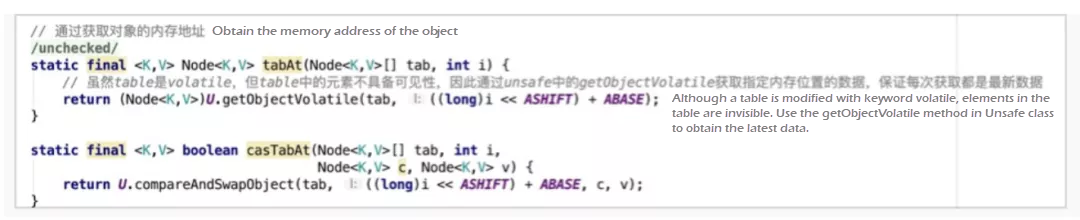Let's move to the logic of the `put()` method. When a put element exists in the hash bucket array and is not in the scaling state, use the keyword synchronized to lock the first element f (head node 2) in the position i in the hash bucket array. Then, double check which one is similar to the DCL singleton pattern. After checking, traverse the elements in the current conflict link, and put the element at an appropriate position. `ConcurrentHashMap` also uses the solution for hash conflicts in HashMap, namely, linked list and R-B tree. The keyword synchronized locks the head node only when hash conflicts occur. This lock is a finer-grained lock than the segment lock. Only one hash bucket is locked in a specific scenario, reducing the impact scope of the lock.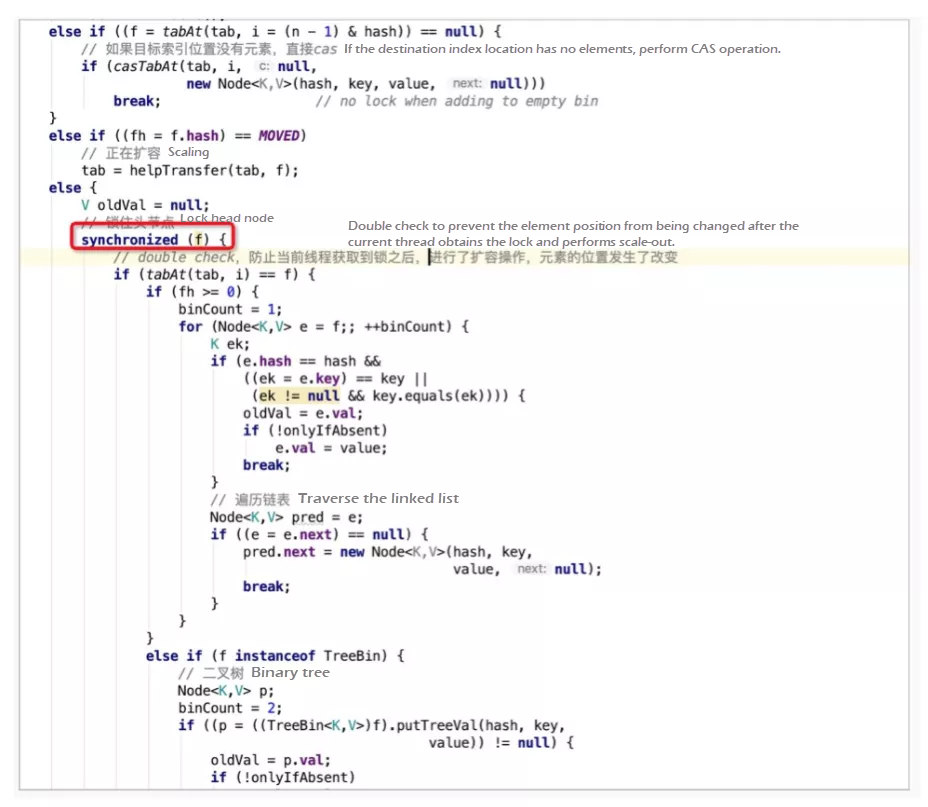For the evolution of solutions in concurrency scenarios, Java Map provides an evolution history from pessimistic locks to optimistic locks and from coarse-grained locks to fine-grained locks. This can also be used as a guideline in our daily concurrent programming.

### 5.3 Concurrent Summation

CounterCell is a useful tool introduced in JDK 1.8 for concurrent summation. In earlier JDK versions, the strategy of trying to sum without lock and retrying to lock in conflict is used. The comments on CounterCell show that it is derived from LongAdder and Striped64. The summation process is listed below:

1. Take `baseCount` as the initial value
2. Traverse each cell in the CounterCell array
3. Accumulate the values of each cell

The `@sun.misc.Contender` annotation was introduced in Java 8 to solve the problem of pseudo sharing among cache lines. What is pseudo sharing? In short, considering the huge difference in speed between CPU and main memory, L1, L2, and L3 caches are introduced into the CPU. The storage unit in cache is cache line, and the size of the cache line is an integer power of 2 in bytes, ranging from 32 bytes to 256 bytes. Most commonly, the size is 64 bytes. Therefore, variables with a size smaller than 64 bytes will share the same cache line. The failure of one variable will affect other variables in the same cache line, resulting in performance degradation. This is the pseudo sharing problem. Considering the differences of cache line units for different CPUs, Java 8 shields the differences through the `@sun.misc.Contender` annotation and fills in variables based on the cache line size. By doing so, the modified variable can exclusively occupy one cache line.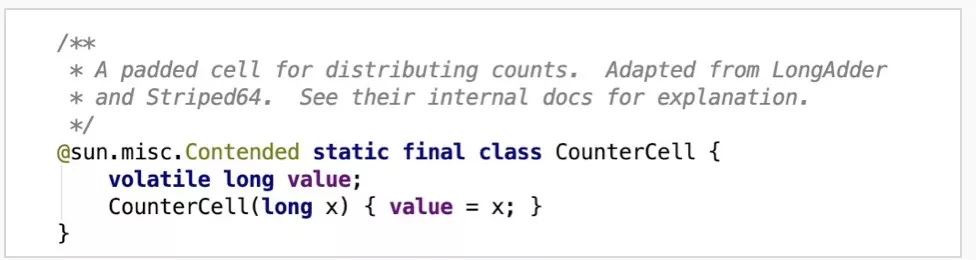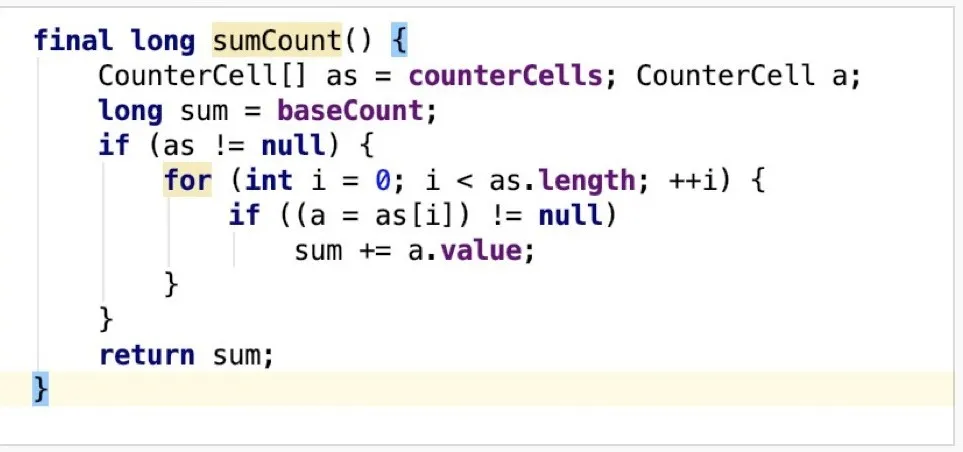Next, let's check out how CounterCell performs count operation. `addCount` is called to count once the capacity in the map changes. The core logic is listed below:

• If `counterCells` is not null, or counterCells is null, and the CAS operation on `baseCount` fails, the subsequent counting processing logic takes effect. Otherwise, perform the CAS operation on `baseCount` until it is succeeded and return the result.
• In subsequent counting processing logic, `fullAddCount`, the core counting method, is called. At least one of the following four conditions must be met to call the method:
1. `counterCells` is empty
2. `counterCells` size is 0
3. `counterCell` at the corresponding position of `counterCells` is empty
4. CAS operation fails to update `counterCell` at the corresponding position of `counterCells`. The semantics of these conditions is that, in the current case, concurrent conflicts have occurred or have once occurred during counting, which needs to be resolved using `CounterCell` first. If `counterCells` and the corresponding element have been initialized (condition 4), the system performs CAS operation for updates first. If it fails, the system calls the `fullAddCount` method to continue processing. If `counterCells` and the corresponding element are not initialized (conditions 1, 2, and 3), AddCount is called for subsequent processing.
• `ThreadLocalRandom.getProbe()` is used as the hash value to determine the cell subscript. This method returns the value of the `threadLocalRandomProbe` field in the current thread. When hash value conflict occurs, the `advanceProbe` method can change the hash value. This is different from the hash value calculation logic in `HashMap` because HashMap needs to ensure that the hash values of the same key after multiple calculations remain the same and can be located. Even if the hash values of two keys conflict with each other, the values cannot be changed casually. The only way to deal with conflicts is to use linked lists or R-B trees. However, in counting scenarios, the key-value relationship requires no maintenance. Instead, an appropriate position in `counterCells` is required to put the counting cell. The difference in positions has no effect on the final sum result. Therefore, when a conflict occurs, a hash value can be changed based on the random policy to avoid the conflict.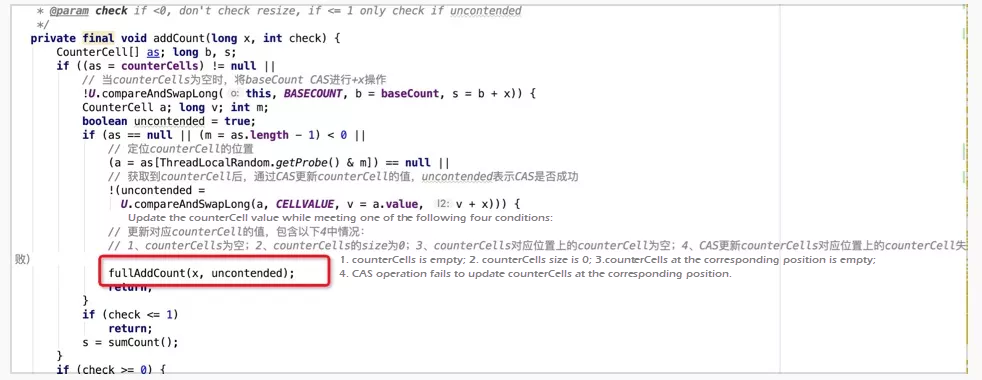Now, let's learn the core calculation logic: `fullAddCount`. The core process is implemented through an endless loop, and the loop body contains three processing branches which are defined as A, B, and C in this article.

• A indicates that the initialization of `counterCells` has been completed. Therefore, the existing `CounterCell` can be updated, and the new CounterCell can be created in the corresponding position.
• B indicates that the initialization of `counterCells` is not completed and no conflict exists. The `cellsBusy` lock is obtained. Then, lock and initialize `counterCells` with an initial capacity of 2.
• C indicates that the initialization of `counterCells` is not completed and a conflict exists. The `cellsBusy` lock is not obtained. Then, perform CAS operation to update `baseCount`, which is included in the final result during summation. This is equivalent to a fallback policy. Since `counterCells` is locked by other threads, the current thread does not need to wait any longer. It can use `baseCount` for accumulation.

The operations involved in the A branch can be split into the following parts:

• a1: The `CounterCell` at the corresponding position has not been created. If the `CounterCell` creation fails using the policy of lock + double-check, continue and retry the creation. The lock used here is the `cellsBusy` lock, which ensures that the `CounterCell` creation and its placement into `counterCells` are performed sequentially. This avoids repeated creation. Additionally, the DCL singleton pattern is used. The `cellsBusy` lock is used to create or scale out `CounterCells`.
• a2: Conflict Detection – The `wasUncontended` variable is input by `addCount`, indicating that the CAS operation for cell update fails and conflicts exist. The hash value [a7] needs to be changed for retrying.
• a3: The `CounterCell` at the corresponding position is not null. Perform CAS operation to update the cell.
• a4:

• Conflict Detection – If the reference value of `counterCells` is not equal to or corresponding to the current thread, another thread has changed the reference of `counterCells`, resulting in a conflict. To solve this problem, set collide to false to scale-out `counterCells` at the next iteration.
• Capacity Limit – The maximum `counterCells` capacity is greater than or equal to the smallest integer power of 2 of the actual CPU core number. When the capacity limit is reached, subsequent scale-out branches will never be executed. The limit shows that the actual concurrency is determined by the CPU cores. When the `counterCells` capacity is equal to the number of CPU cores, ideally, even if all CPU cores are running different counting threads at the same time, there should be no conflict. Each thread should select its own cell for processing. If a conflict occurs, it must be a problem with the hash value. Therefore, the solution is to recalculate the hash value a7, rather than scaling out `counterCells`. This way, unnecessary wastes of storage space can be avoided; great!
• a5: Update the scale-out flag, indicating that `counterCells` will be scaled out in the next iteration
• a6: Obtain the cellsBusy lock and double the capacity each time
• a7: Change the hash value
``````private final void fullAddCount(long x, boolean wasUncontended) {
int h;
// Initialize the probe
if ((h = ThreadLocalRandom.getProbe()) == 0) {
wasUncontended = true;
}
// Control scale-out
boolean collide = false;                // True if last slot is non-empty
for (;;) {
CounterCell[] as; CounterCell a; int n; long v;
// 【A】counterCells is initialized
if ((as = counterCells) != null && (n = as.length) > 0) {
// 【a1】CounterCell at the corresponding position is not created
if ((a = as[(n - 1) & h]) == null) {
// cellsBusy is a lock and cellsBusy = 0 indicates no conflict
if (cellsBusy == 0) {            // Try to attach new Cell
// Create new CounterCell
CounterCell r = new CounterCell(x); // Optimistic create
// Double check and lock（perform CAS operation to set cellsBusy to 1）
if (cellsBusy == 0 &&
U.compareAndSwapInt(this, CELLSBUSY, 0, 1)) {
boolean created = false;
try {               // Recheck under lock
CounterCell[] rs; int m, j;
// Double check
if ((rs = counterCells) != null &&
(m = rs.length) > 0 &&
rs[j = (m - 1) & h] == null) {
// Put the new CounterCell into counterCells
rs[j] = r;
created = true;
}
} finally {
// Unlock. Why CAS operation is not performed? It is because operations in the process are executed in sequence with the lock obtained. So, no concurrent update issue exists.
cellsBusy = 0;
}
if (created)
break;
// Retry if creation fails
continue;           // Slot is now non-empty
}
}
// cellsBusy is not 0, indicating that other threads obtained the lock. Scale-out cannot be performed.
collide = false;
}
//【a2】Detect conflicts
else if (!wasUncontended)       // CAS already known to fail
// CAS operation performed by addCount for updating the cell is failed and conflict occurs. Retry CAS operation.
wasUncontended = true;      // Continue after rehash

//【a3】CounterCell at the corresponding position is not empty. Perform CAS operation for update.
else if (U.compareAndSwapLong(a, CELLVALUE, v = a.value, v + x))
break;
//【a4】Capacity limit
else if (counterCells != as || n >= NCPU)
// The maximum counterCells capacity is greater than the smallest integer power of 2 of the actual CPU core number.
// The limit shows that the actual concurrency is determined by the CPU cores. When the counterCells capacity is equal to the number of CPU cores, ideally, even if all CPU cores are running different counting threads at the same time, there should be no conflict. Each thread should select its own cell for processing. If a conflict occurs, it must be a problem with the hash value. The solution is to re-calculate the hash value by using h = ThreadLocalRandom.advanceProbe(h), instead of scale-out.

// If n is larger than the CPU core number, the subsequent branches will not be executed.
collide = false;            // At max size or stale
// 【a5】Update the scale-out flag
else if (!collide)
// Indicate that the mapped position in cell is not empty and the CAS operation for update is failed. Conflict occurs. Set collide to false, indicating that scale-out is performed in the next iteration to reduce competition.
// If competition exists， perform rehash and scale out. If the capacity is larger than the CPU core number, perform rehash only.
collide = true;
// 【a6】Obtain the lock and perform scale-out
else if (cellsBusy == 0 &&
U.compareAndSwapInt(this, CELLSBUSY, 0, 1)) {
// Obtain the lock and perform scale-out
try {
if (counterCells == as) {// Expand table unless stale
// Double the capacity
CounterCell[] rs = new CounterCell[n << 1];
for (int i = 0; i < n; ++i)
rs[i] = as[i];
counterCells = rs;
}
} finally {
cellsBusy = 0;
}
collide = false;
continue;                   // Retry with expanded table
}
//【a7】Change the hash value
}
// 【B】The counterCells initialize is not completed and no conflict exists. Lock and initialize counterCells.
else if (cellsBusy == 0 && counterCells == as &&
U.compareAndSwapInt(this, CELLSBUSY, 0, 1)) {
boolean init = false;
try {                           // Initialize table
if (counterCells == as) {
CounterCell[] rs = new CounterCell;
rs[h & 1] = new CounterCell(x);
counterCells = rs;
init = true;
}
} finally {
cellsBusy = 0;
}
if (init)
break;
}
// 【C】The counterCells initialization is not completed and conflict exists. Perform CAS operation to update baseCount.
else if (U.compareAndSwapLong(this, BASECOUNT, v = baseCount, v + x))
break;                          // Fall back on using base
}``````

The design of `CounterCell` is very clever. It is derived from LongAdder in JDK 1.8. The core idea is to use `baseCount` for accumulation in scenarios with low concurrency. Otherwise, use `counterCells` to hash different threads into different cells for calculation and ensure the isolation of accessed resources as much as possible to reduce conflicts. Compared with the CAS strategy in `AtomicLong`, LongAdder can reduce the number of CAS retries and improve the calculation efficiency in high concurrency scenarios.

## 6. Conclusion

The sections above cover a small part of the source codes of Java Map. However, they include most of the core features and cover most of the daily development scenarios. Reading source codes is an interesting endeavor, it allows you to better understand the thought process of the code developer. The process of transforming information into knowledge and applying it is painful but pleasurable as well.

0 0 0
Share on

# Related Products

• ##Machine Learning Platform For AI

An end-to-end platform that provides various machine learning algorithms to meet your data mining and analysis requirements.

• ##Epidemic Prediction Solution

This technology can be used to predict the spread of COVID-19 and help decision makers evaluate the impact of various prevention and control measures on the development of the epidemic.

• ##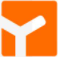YiDA Low-code Development Platform

A low-code development platform to make work easier

• ##mPaaS

Help enterprises build high-quality, stable mobile apps